# Experimental Investigations on Conventional Solar Still with Sand Heat Energy Storage

Experimental Investigations on Conventional Solar Still with Sand Heat Energy Storage

Ravishankar SathyamurthyP.K. Nagarajan M. Edwin B. Madhu S.A. El-Agouz A. Ahsan D. Mageshbabu

Department of Mechanical Engineering, S.A. Engineering College, Chennai-600077

University College of Engineering, Nagercoil, Kanyakumari, Tamil Nadu, India

Mechanical and Power Engineering, Tanta University, Egypt

Department of Civil Engineering, University Putra Malaysia, Serdong

Corresponding Author Email:
raviannauniv23@gmail.com
Page:
597-603
|
DOI:
https://doi.org/10.18280/ijht.340407
|
Accepted:
|
Published:
31 December 2016
| Citation

OPEN ACCESS

Abstract:

In the present investigation, a detailed experimental study is carried out on a conventional single slope solar still with sand heat energy storage for improving the yield of fresh water. Yield of the solar still depends on the type of energy storage material used in the basin. Yield of the present study is compared with the solar still without any storage medium. Similarly, mathematically correlations of yield as a function of water and basin temperature were depicted. The results show that the use of sand as energy storage in cuboidal boxes the yield of solar still has improved by 145% than that of conventional single slope solar still. The total yield from the solar still with and without energy storage materials was found to be 5.1 and 1.9 kg/m2day respectively.

Keywords:

Solar still, Desalination, Energy storage, Sand, Cuboidal box.

1. Introduction

Solar energy is widely used in various applications such as collector based heating and cooling system, power generation, combined PV/T systems, desalination -. Solar still is the oldest and traditional method of getting fresh drinking water in the coastal area. Ground water near the coastal areas is majorly affected by the sea water which has high saline content -. Researchers identified that not only in the coastal area but upto 60-80 kms surrounding ground water is affected. Due to the lower yield solar still is not widely used and many researches are still going on to improve the yield of fresh water -.

Nagarajan et al.  theoretically analyzed the effect of forcing the air over the cover of a triangular pyramid solar still. The optimum velocity of air over the condensing glass surface is one of the important parameter in improving the yield of fresh water. The yield is higher with an optimum velocity of 4.5 m/s on the coastal areas of Tamil Nadu, India and the optimum water mass inside the basin of 20 kg.

Sathyamurthy et al.  experimentally investigated a triangular pyramid solar still with latent heat energy storage. Results from their study shows that the use of latent heat energy storage improves the yield from 4.2 to 4.8 kg/m2day. Also, the mass of phase change material and water plays a vital role in improving the yield of fresh water. Sathyamurthy et al.  theoretically analyzed the effect of baffles in inclined solar still. Theoretical studies show that the effect of baffles increases the exposure area of water and improves the evaporation. Based on the study a new empirical relation of Nusselt number and heat transfer coefficient are arrived with an RHN model. Similarly, for improving the yield of fresh water semi circular trough absorber solar still cum solar water heater has been identified. Results show that a low cost cheap material as absorber and trough with baffles improves the yield of fresh water and water temperature. The higher water temperature can be utilized by coupling it to another solar still for better evaporation with minimal wastage. Harris Samuel et al.  experimentally investigated the use of salt heat energy storage in a conventional single slope solar still. Similarly, the yield of solar still is compared with a conventional solar still without modification and with sponges. The results show that the use of sponge material affect the quality of fresh water and frequent replacement of the material has increased the cost of fresh water produced. Naveen kumar et al.  theoretically studied the integration of inclined solar still with triangular pyramid solar still. Results show that the improvement in yield completely depends on the temperature of water and the incoming solar energy received by the collector and the solar still.

Even though Omara and Kabeel et al.  investigated the use of black and yellow sand as absorber bed material of conventional solar still the yield are found to be 4 and 3 kg/m2 day respectively. Results show that the maximum hourly yield from the solar still was 0.5 and 0.6 kg/m2 while using yellow and black sand respectively. Similarly, the yield decreases with an increase in the depth (height) of sand material. Also, the percentage improvement in yield of solar still using black sand is higher than that of yellow sand as bed material.

In the present study, sand material in cuboidal boxes with a specified dimension is taken as sensible heat energy storage for improving the yield of fresh water.

2. Experimental Setup

## 1.png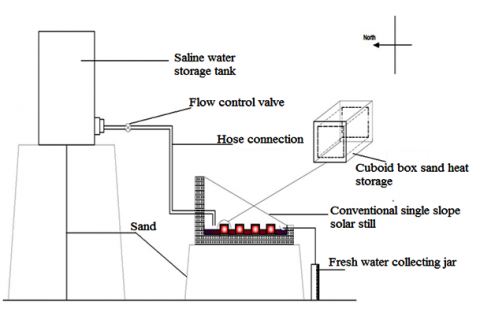Figure 1. Schematic diagram of conventional solar still with cuboid box sand heat energy storage

## 2.pngFigure 2. Photograph of the experimental setup

Figure 1 shows the schematic diagram of a conventional single slope solar still with cuboid box (top side open) as sand heat storage. The basin length and breadth are 1 m x 1 m. Sand is one of the excellent heat storage medium commonly used. As it is excellent sensible heat storage, it is taken in mild steel cuboid boxes of 0.1 m x 0.1 m x 0.1 m dimensions (Figure 2). Each sand box were placed at a distance of 0.1 m from each other and yellow sand is used as energy material. Also, each cuboid box placed in the basin increases the surface area of water. Each box is filled with 0.5 kg of sand material and a total of 15 boxes are kept in the solar still with final increased water exposure area of 0.85 m2. Temperature of different element of solar still are measured using a RTD sensor with an error of +/- 1oC. Using a calibrated beaker the distillate collected in every hour is measured. Environmental parameters such as solar intensity and wind velocity are measured using an solar power meter and 3 cup anemometer respectively. The detailed error and experimental uncertainty are discussed in Table. 1. A constant water depth of 0.02 m is maintained in the solar still and water is fed into the basin. The equivalent water mass with increased exposure area of water in the solar still is found as 17 kg (dw=0.02 m and Aw=0.85 m2). The experiments are carried out in the North-South orientation while the glass is inclined facing the south direction. The glass of the solar still is inclined at an angle of 13o coinciding with horizon. Experiments were conducted in a domestic region of Chennai, India from 7 AM to 12 AM and usually carried out on a bright and sunny condition. The variation of wind velocity over the period has vast deviation throughout the year and only the average values of wind velocity is taken into consideration.

Table. 1. Accuracy, range and errors for measuring instruments

 Instrument Accuracy Range Error (%) Thermocouple ±1oC 0-100oC 0.25 Solar power  meter ±1W/m2 0-2500 W/m2 2.5 Anemometer ±0.1m/s 0-45 m/s 10 Beaker ±10mL 0-1000mL 10

Table. 2. Observed error and standard uncertainity

 Instrument Observed error (%) Standard Unertainity Thermocouple 1.2 ±0.57 oC Solar power  meter 3.1 ±0.57 W/m2 Anemometer 6.8 ±0.05 m/s Beaker 8.3 ±5.77 mL
3. Data Reduction

3.1 Conventional solar still with and energy storage material

3.1.1 Energy balance on basin surface

The basin temperature (Tb) is determined as ,

$I(t) \tau_{\mathrm{g}} \tau_{\mathrm{w}} \alpha_{\mathrm{b}}=h_{1}\left(T_{b}-T_{w}\right)+U_{b}\left(T_{b}-T_{a}\right)$(1)

$T_{b}=\frac{I(t) \tau_{\mathrm{g}} \tau_{\mathrm{w}} \alpha_{\mathrm{b}}+h_{1} T_{w}+U_{b} T_{a}}{h_{1}+U_{b}}$(2)

h1= 109 W/m2K  and the overall heat transfer coefficient Ub is given as ,

$\frac{1}{U_{b}}=\frac{1}{h_{c, g-a}}+\frac{L_{1}}{k_{1}}+\frac{1}{h_{c, b-w}}$(3)

3.1.2 Energy balance on water surface

The temperature of water (Tw) is determined as ,

$T_{w}=\frac{f(t)}{a}\left[1-e^{\frac{-a t}{M_{e q u}}}\right]+T_{w, j}\left[e^{\frac{-a t}{M_{e q u}}}\right]$(4)

where “f (t)” and “a” values are determined as mentioned in Appendix A.1.1.

$M_{e q u}=m_{w} \bullet C_{p w}+m_{e s} \bullet C_{p e s}$(5)

While for the solar still without energy storage material the equivalent water mass is determined as,

$M_{e q u}=m_{w} \bullet C_{p w}$(6)

The specific heat capacity of saline water (Cpw) is given by ,

$C_{p w}=s_{1}+s_{2} T_{w}+s_{3} T_{w}^{2}+s_{4} T_{w}^{3}$(7)

where, s1, s2, s3 and s4value are appended in Appendix A.1.2.

3.1.3 Energy balance on glass surface

The glass temperature (Tg) is determined as,

$I(t) \alpha_{g}=h_{3}\left(T_{g}-T_{a}\right)-h_{2}\left(T_{w}-T_{g}\right)$(8)

$T_{g}=\frac{I(t) \alpha_{g}+h_{2} T_{w}+h_{3} T_{a}}{h_{2}+h_{3}}$(9)

where,

$h_{2}=h_{c, w-g}+h_{e, w-g}+h_{r, w-g}$(10)

3.1.4 Estimation of yield from solar still

Yield of fresh water (mew) from the solar still is calculated as ,

$m_{e w}=\frac{h_{e w-g} A_{w}\left(T_{w}-T_{g}\right)}{h_{f g}}$(11)

4. Results and Discussion

4.1 Conventional single slope solar still

## 3.png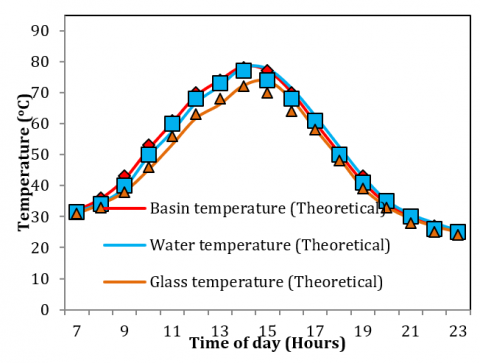Figure 3. Theoretical and experimental hourly variation of basin, water and glass temperature of conventional solar still

Figure 3 and 4 shows the hourly variation of theoretical and experimental variation of basin, water, glass temperatures, yield and solar intensity during the experimental day. It can be seen that the temperature of water in the case of conventional solar still without any storage has lesser temperature as compared to solar still with sand heat energy storage. Even though the same equivalent water mass is maintained inside the basin the maximum water temperature and yield are found as 75oC and 0.5 kg/m2hr respectively and normally 28% and 45% lesser than solar still with sand heat energy storage.

## 4.png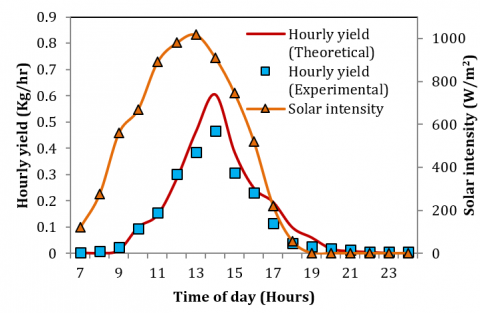Figure 4. Hourly variation yield (theoretical and experimental) and solar intensity of conventional solar still

4.2 Conventional single slope solar still with cuboidal box sand heat energy storage

## 5.png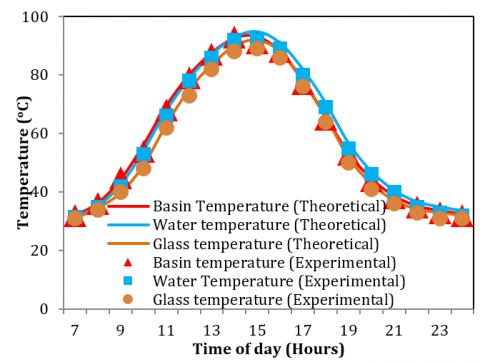Figure 5. Theoretical and experimental hourly variation of basin, water and glass temperature of conventional solar still with sand heat energy storage

Figure 5 and 6 shows the hourly variation of theoretical and experimental variation of basin, water, glass temperatures, yield and solar intensity during the experimental day. It can be observed that the maximum temperature of water inside the solar still while using sand as heat storage material in cuboidal boxes is 87oC and that is 2.5% higher than using salt heat storage in encapsulated balls. Similarly the deviations between experimental and theoretical values are found as 4.3%. The maximum hourly yield for theoretical and experimental values is found as 0.7 and 0.6 kg/m2 and similar to encapsulated ball salt heat storage. Due to the higher heat storage in sand during the off shine period the yield is higher than the previous method.

Figure 7 (a) and (b) shows the variation of water and basin temperature with respect to solar intensity using sand heat storage. It can be seen that the water and basin temperature are exactly matching and the maximum temperature of water and basin are observed to be 90 and 92oC respectively with a solar intensity of 1050 W/m2. The characteristic equation using 2nd order polynomial equation has been derived using the experimental values of water and basin temperature and solar intensity.

## 6.png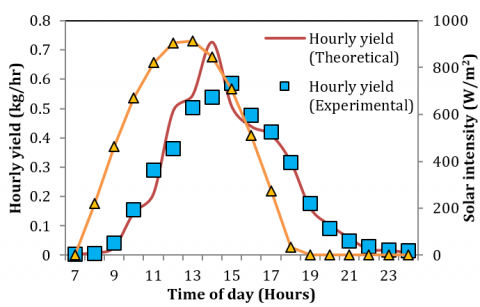Figure 6. Hourly variation yield (theoretical and experimental) and solar intensity of conventional     solar still with sand heat energy storage

## 7a.png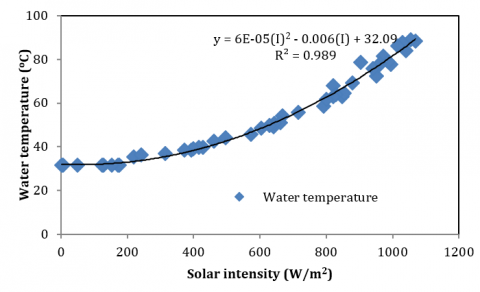Figure 7(a). Variation of water temperature with respect to solar intensity using sand heat storage

## 7b.pngFigure 7(b). Variation of basin temperature with respect to solar intensity using sand heat storage

The characteristic equation for water temperature is found as,

$T_{w}=6 \times 10^{-5}(I)^{2}-0.006(I)+32.09$(1)

and the characteristic equation for basin temperature is found as,

$T_{b}=5 \times 10^{-5}(I)^{2}+32.07$(2)

## 8a.pngFigure 8 (a). Variation of yield from solar still with respect to water temperature using sand heat storage

## 8b.png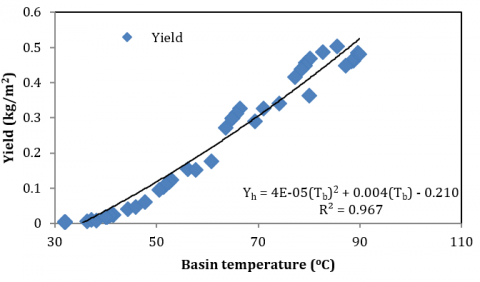Figure 8 (b). Variation of yield from solar still with respect to basin temperature using sand heat storage

Figure 8 (a) and (b) shows the variation of yield from solar still as a function of water and basin temperature. It is clearly evident that the yield from both the cases is similar and the coefficient of regression is R2=0.967. The characteristic equation of yield as a function of water temperature and basin temperature are found as,

$Y_{h}=2 \times 10^{-5}\left(T_{w}\right)^{2}+0.006 T_{w}-0.256$(3)

$Y_{h}=4 \times 10^{-5}\left(T_{b}\right)^{2}+0.004 T_{b}-0.210$(4)

Figure 9 shows the theoretical and accumulated yield from solar still using sand heat storage. The average yield from the present solar still is 5 kg/m2 during the months of April, May, June (summer conditions). Whereas, the average yield from solar still during the months of January, February and March (winter conditions) is found as 4.1 kg/m2. The deviation between theoretical and experimental value is found as 8% and 3.2% during winter and summer respectively.

5. Conclusions

From the above study, following conclusions are arrived.

• The yield of solar still with and without energy storage material are found as 5 and 1.9 kg/m2day.
• Deviations between theoretical and experimental studies of accumulated yield are found as 12%.
• Selection of sensible heat storage material plays an important role in improving the yield of fresh water solar still. Lower the specific heat leads to higher heat gain and continuous rejection of heat into the water for continuous and rapid evaporation from the free surface.
• Similarly, the effective temperature difference between water and glass improves the yield of solar still. While analyzing the yield of solar still with energy storage the temperature difference improves by 80% as compared to the solar still without any heat storage.

## 9.png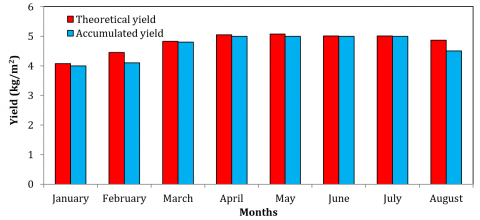Figure 9. Variation of theoretical and accumulated yield from modified solar still during experiments

Nomenclature

A      Area (m2)

C      Specific heat capacity (J/kg K)

h       heat transfer Co-efficient (W/m2 K)

hfg    latent heat of vaporization (J/kg)

k       thermal conductivity (W/m2 K)

t        time step (s)

U      overall heat transfer coefficient (W/m2 K)

Y      salinity (g/kg)

Greek symbols

α       absorptivity

τ       Transmissivity

μ       Dynamic viscosity (m2/s)

ν       Kinematic viscosity ( kg/ms)

ρ       Density (kg/m3)

σ       Stefen Boltzman Constant (5.67 x 10-8 W/m2K4)

ε       emissivity

θ       Inclination angle (degrees)

Subscripts

a       air

atm  atmosphere

b       basin

c       convection

e       evaporation

es     energy storage

equ  equivalent

g       glass

w      water

APPENDIX A

A.1.1. Estimation of variable f(t) and a

The values of f(t) and a are estimated using ,

$f(t)=I^{\prime}(t) \tau_{g} \alpha_{w}+\left[\frac{I^{\prime}(t) \tau_{g} \tau_{w} \alpha_{b}+U_{b} T_{a}}{\left(1+\frac{U_{b}}{h_{1}}\right)}\right]+\left[\frac{I^{\prime}(t) \alpha_{g}+h_{r g s} T_{s}+h_{c g a} T_{a}}{\left(1+\frac{h_{3}}{h_{2}}\right)}\right]$

$a=\left[\frac{h_{1} U_{b}}{h_{1}+U_{b}}\right]+\left[\frac{h_{2} h_{3}}{h_{2}+h_{3}}\right]$

A.1.2. Estimation of value of constant variable s1, s2, s3 and s4 for determining specific heat capacity of saline water

The values of s1, s2,s3 and s4 are calculated using ,

C      The seawater specific heat at constant pressure

CP=J/kg K

$s_{1}=4206.8+6.6197 Y+1.2288 \times 10^{-2} Y^{2}$

$s_{2}=-1.1262+5.4178 \times 10^{-2} Y-2.2719 \times 10^{-6} Y^{2}$

$s_{3}=1.2026 \times 10^{-2}-5.5366 \times 10^{-4} Y+1.8906 \times 10^{-6} Y^{2}$

$s_{4}=6.8874 \times 10^{-7}+1.517 \times 10^{-6} Y-4.4268 \times 10^{-9} Y^{2}$

where Y is the salinity level in water.

References

 Arunkumar, T., Denkenberger, D., Velraj, R., Sathyamurthy, R., Tanaka, H. and Vinothkumar, K., “Experimental study on a parabolic concentrator assisted solar desalting system,” Energy Conversion and Management, vol. 105, pp. 665-674, 2015. DOI: 10.1016/j.enconman.2015.08.021.

 Sathyamurthy, R., El-Agouz, S.A., Nagarajan.P.K, Arunkumar, T, Subramani, J., Mageshbabu. D., Madhu. B., Bharadwaaj. R. and Prakash, N., “A review of integrating solar collectors to solar still,” Renewable and Sustainable Energy Reviews, Dec 2016. DOI: 10.1016/j.rser.2016.11.223.‘’

 Nagarajan, P. K., El-Agouz, S. A., Harris Samuel, Edwin, M., Madhu. B., Mageshbabu. D.,  Sathyamurthy, R. and Bharathwaaj, R. “Analysis of an inclined solar still with baffles for improving the yield of fresh water.” Process Safety and Environmental Protection, vol. 105, pp. 326-337, 2016. DOI:  10.1016/j.psep.2016.11.018.‘’

 A.E. Kabeel, T. Arunkumar, D.C. Denkenberger, Ravishankar Sathyamurthy. “Performance enhancement of solar still through efficient heat exchange mechanism- A review,” 2016. DOI: 10.1016/j.applthermaleng.2016.12.044.

 Arunkumar, T., Velraj, R., Ahsan, A., Khalifa, A. J. N., Shams, S., Denkenberger, D. and Sathyamurthy, R., “Effect of parabolic solar energy collectors for water distillation,” Desalination and Water Treatment, pp. 1-9, 2015. DOI: 10.1080/19443994.2015.1119746.

 Arunkumar, T., Velraj, R., Denkenberger, D. C., Sathyamurthy, R., Kumar, K. V. and Ahsan, A., “Productivity enhancements of compound parabolic concentrator tubular solar stills,” Renewable Energy, vol. 88, pp. 391-400, 2016. DOI: 10.1016/j.renene.2015.11.051.

 Arunkumar, T., Velraj, R., Denkenberger, D., Sathyamurthy, R., Vinothkumar, K., Porkumaran, K. and Ahsan, A., “Effect of heat removal on tubular solar desalting system,” Desalination, vol. 379, pp.24-33, 2016. DOI: 10.1016/j.desal.2015.10.00.

 El-Maghlany, W. M., El-Samadony, Y. A. F. and Kabeel, A. E., “Glass cover inclination angle effect on the radiation shape factor within conventional solar still,” Desalination and Water Treatment, vol. 57, no. 38, 17722-17730, 2016. DOI: 10.1080/19443994.2015.1102090.

 Sharshir, S. W., Peng, G., Yang, N., El-Samadony, M. O. A. and Kabeel, A. E., “A continuous desalination system using humidification–dehumidification and a solar still with an evacuated solar water heater,” Applied Thermal Engineering, vol. 104, pp. 734-742, 2016. DOI: 10.1016/j.applthermaleng.2016.05.120.

 Omara, Z. M., Kabeel, A. E., Abdullah, A. S. and Essa, F. A. “Experimental investigation of corrugated absorber solar still with wick and reflectors,” Desalination, vol. 381, pp. 111-116, 2016. DOI: 10.1016/j.desal.2015.12.001.

 Harris Samuel, D. G., Nagarajan, P. K., Arunkumar, T., Kannan, E. and Sathyamurthy, R., “Enhancing the solar still yield by increasing the surface area of water—A review,” Environmental Progress & Sustainable Energy, vol. 35, no. 3, pp. 815-822, 2016. DOI: 10.1002/ep.12280.

 Nagarajan, P. K., El-Agouz, S. A., Arunkumar, T. and Sathyamurthy, R., “Effect of forced cover cooling technique on a triangular pyramid solar still,” International Journal of Ambient Energy, pp. 1-8, 2016. DOI: 10.1080/01430750.2016.1159609.

 Nagarajan, P. K., Subramani, J., Suyambazhahan, S. and Sathyamurthy, R., “Nanofluids for solar collector applications: a review,” Energy Procedia, vol. 61, pp. 2416-2434, 2014. DOI: 10.1016/j.egypro.2014.12.017.

 Murugesan, V., Seralathan, S. and Ravishankar, S., “Theoretical studies of an improved inclined solar still with baffle plates,” International Journal of Applied Engineering Research (IJAER), vol. 10, no. 13, pp. 11439-11444, 2015.

 Ravishankara, S., Nagarajan, P. K., Vijayakumar, D. and Jawahar, M. K., “Phase change material on augmentation of fresh water production using pyramid solar still,” International Journal of Renewable Energy Development, vol. 2, no. 3, pp. 115, 2013. DOI: 10.14710/ijred.2.3.115-120.

 Sathyamurthy, R., Harris Samuel, D. G., Nagarajan, P. K. and Arunkumar, T., “Geometrical variations in solar stills for improving the fresh water yield—A review,” Desalination and Water Treatment, pp. 1-15, 2016. DOI: 10.1080/19443994.2015.1136241.

 Sathyamurthy, R., Nagarajan, P. K. and Vijayakumar, D., “Experimental validation of fresh water production using triangular pyramid solar still with PCM storage,” International Journal of Engineering Research in Africa, vol. 20, pp. 51-58, 2016. DOI: 10.4028/www.scientific.net/JERA.20.51.

 Sathyamurthy, R., Samuel, D. H. and Nagarajan, P. K., “Theoretical analysis of inclined solar still with baffle plates for improving the fresh water yield,” Process Safety and Environmental Protection, vol. 101, pp. 93-107, 2016. DOI: 10.1016/j.psep.2015.08.010.

 Sathyamurthy, R., Samuel, D. H., Nagarajan, P. K. and El-Agouz, S. A., “A review of different solar still for augmenting fresh water yield,” Journal of Environmental Science and Technology, vol. 8, no. 6, 244-265, 2015. DOI: 10.3923/jest.2015.244.265.

 Sathyamurthy, R., Nagarajan, P. K., El-Agouz, S. A., Jaiganesh, V. and Khanna, P. S., “Experimental investigation on a semi-circular trough-absorber solar still with baffles for fresh water production,” Energy Conversion and Management, vol. 97, pp. 235-242, 2015. DOI: 10.1016/j.enconman.2015.03.052.

 Sathyamurthy, R., El-Agouz, S. A. and Dharmaraj, V., “Experimental analysis of a portable solar still with evaporation and condensation chambers,” Desalination, vol. 367, pp. 180-185, 2015. DOI: 10.1016/j.desal.2015.04.012.

 Sathyamurthy, R., Samuel, D. H., Nagarajan, P. K. and Jaiganesh, V., “Experimental investigation of a semi circular trough solar water heater,” Applied Solar Energy, vol. 51, no. 2, pp. 94-98, 2015. DOI: 10.3103/S0003701X15020152.

 Sathyamurthy, R., Kennady, H. J., Nagarajan, P. K. and Ahsan, A., “Factors affecting the performance of triangular pyramid solar still,” Desalination, vol. 344, pp. 383-390, 2014. DOI: 10.1016/j.desal.2014.04.005.

 Sathyamurthy, R., Nagarajan, P. K., Kennady, H., Ravikumar, T. S., Paulson, V. and Ahsan, A., “Enhancing the heat transfer of triangular pyramid solar still using phase change material as storage material,” Frontiers in Heat and Mass Transfer (FHMT), vol. 5, no. 1, 2014. DOI: 10.5098/hmt.5.3.

 Sathyamurthy, R., Nagarajan, P. K., Subramani, J., Vijayakumar, D. and Ali, K. M. A., “Effect of water mass on triangular pyramid solar still using phase change material as storage medium,” Energy Procedia, vol. 61, pp. 2224-2228, 2014. DOI: 10.1016/j.egypro.2014.12.114.

 Samuel, D. H., Nagarajan, P. K., Sathyamurthy, R., El-Agouz, S. A. and Kannan, E., “Improving the yield of fresh water in conventional solar still using low cost energy storage material,” Energy Conversion and Management, vol. 112, pp. 125-134, 2016. DOI: 10.1016/j.enconman.2015.12.074.

 Naveen Kumar, P., Harris Samuel, D. G., Nagarajan, P. K. and Sathyamurthy, R. “Theoretical analysis of a triangular pyramid solar still integrated to an inclined solar still with baffles,” International Journal of Ambient Energy, pp. 1-7, 2016. DOI: 10.1080/01430750.2016.1181569.

 Omara, Z. M. and Kabeel, A. E., “The performance of different sand beds solar stills,” International Journal of Green Energy, vol. 11, no. 3, pp. 240-254, 2014. DOI: 10.1080/15435075.2013.769881.

 El-Sebaii, A. A., Al-Ghamdi A. A., Al-Hazmi F. S., and Faidah, Adel S. “Thermal performance of a single basin solar still with PCM as a storage medium,” Applied Energy, vol. 86,  no. 7, pp. 1187-1195, 2009. DOI: 10.1016/j.apenergy.2008.10.014.

 Velmurugan, V., Naveen Kumar, K.J., Noorul Haq, T., and Srithar, K. “Performance analysis in stepped solar still for effluent desalination,” Energy, vol. 34, no. 9, 1179-1186, 2009.  DOI: 10.1016/j.energy.2009.04.29.

 Shukla SK, Sorayan VPS. “Thermal modeling of solar stills: an experimental validation,” Renew Energy, vol. 30, pp. 683–99, 2005. DOI: 10.1016/j.renene.2004.03.009.

 Zurigat, Y. H. and Mousa, A.A.K. “Modeling and performance analysis of a regenerative solar desalination unit,” Appl Therm Eng, vol. 24, pp. 1061–1072, 2004. DOI: 10.1016/j.applthermaleng.2003.11.010.